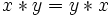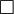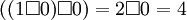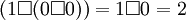# Commutative property

(Redirected from Commutative)
Jump to: navigation, search

In mathematics, the commutative property states that a binary operationon a set A is said to be commutative if for allin A we have. An example of a commutative operation is addition in the set of real numbers. When a group's operation is commutative, it is said to be abelian. If, then the operation is said to be anticommutative.

In layman's terms, an equation demonstrates commutativity when the constants or variables can be moved around an operation without changing the answer (e.g. 1 + 2 = 2 + 1 or 2 * 3 = 3 * 2). It is as if the numbers are "commuting" from one place to another. Thus, using real numbers, both multiplication and addition are commutative, and subtraction and division are not. For vectors, the dot product is commutative, while the cross product is anticommutative.

The commutative property does not imply the associative property, nor vice versa. For example, matrix multiplication is associative but not commutative. Also, define the functionon integers by. Although,is commutative, it is not associative, asbut.